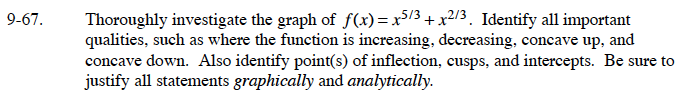### Home > CALC > Chapter 9 > Lesson 9.2.2 > Problem9-67

9-67.$f^\prime(x)=\frac{5}{3}x^{2/3}+\frac{2}{3}x^{-1/3}=\frac{1}{3}x^{-1/3}(5x+2)$

Solve f′(x) = 0 to locate the extrema and determine the increasing/decreasing intervals.

$f^{\prime\prime}(x)=\frac{10}{9}x^{-1/3}+\frac{2}{9}x^{-4/3}=\frac{2}{9}x^{-4/3}(5x-1)$

Solve f''(x) = 0 to determine points of inflection and the intervals of concavity.